Function Repository Resource:

# FaurePoint

Get a point belonging to the Faure sequence

Contributed by: Jan Mangaldan
 ResourceFunction["FaurePoint"][k,d,b] gives the kth d-dimensional point belonging to the base-b Faure sequence. ResourceFunction["FaurePoint"][k,d] automatically chooses the base.

## Details

A Faure sequence is a quasirandom sequence (also called a low-discrepancy sequence) based on the permutation of a van der Corput sequence with a Pascal matrix.
The base should be a prime number greater than or equal to the dimension of the point.
ResourceFunction["FaurePoint"] gives a point belonging to the region [0,1]d.

## Examples

### Basic Examples (2)

A 2D Faure point:

 In:=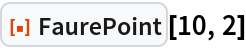Out=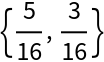A 3D Faure point:

 In:=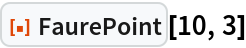Out=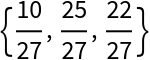### Scope (2)

Use a different base:

 In:=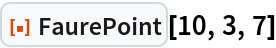Out=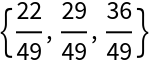In:=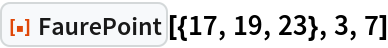Out=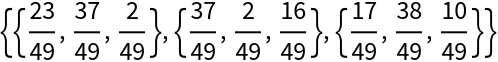### Applications (2)

Show the structure of a Faure sequence in dimension 2:

 In:=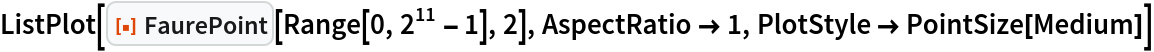Out=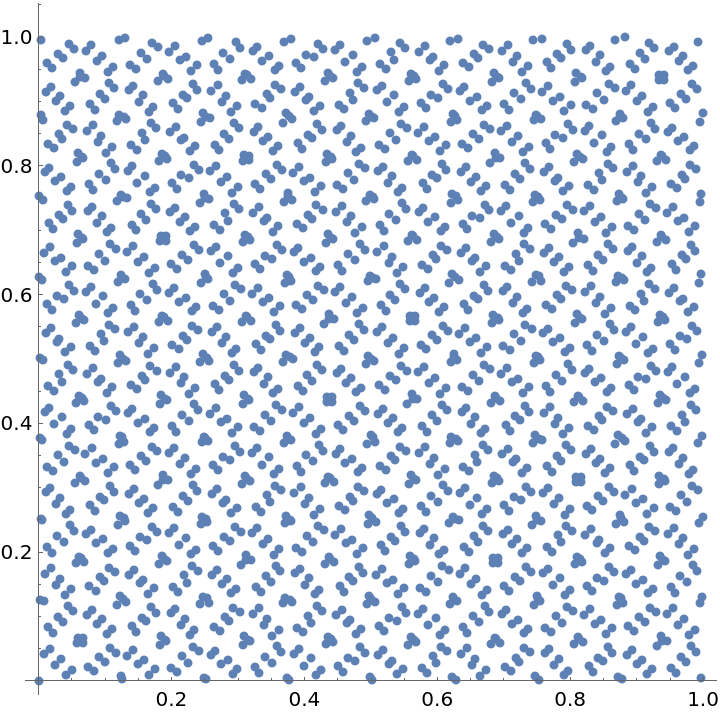Use the Faure sequence to approximate π by quasi-Monte Carlo integration:

 In:=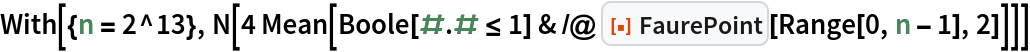Out=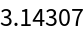### Properties and Relations (2)

Use RescalingTransform to map Faure sequence points to other rectangular or cuboidal domains:

 In:=Out=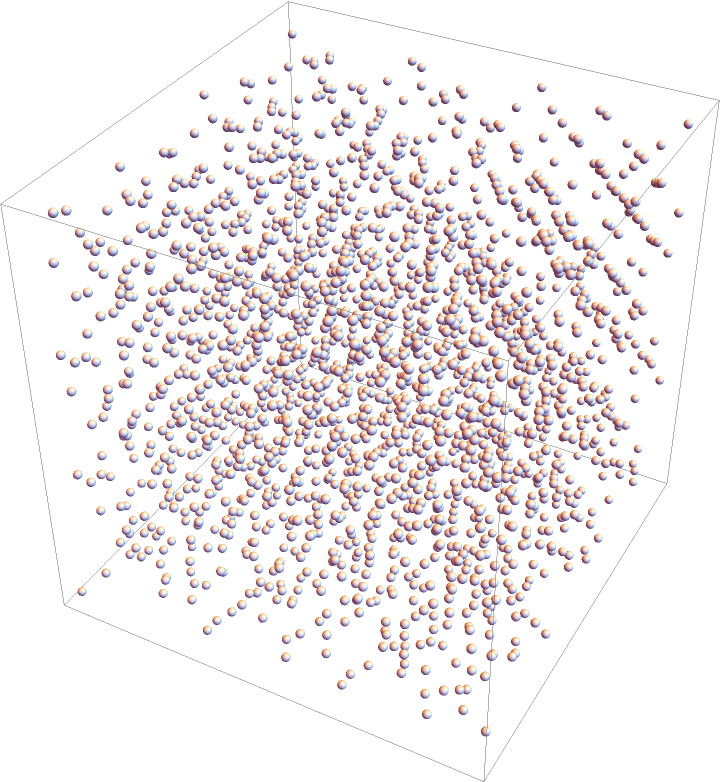If the unit square is divided into rectangles with area b-n, each rectangle contains exactly one member of the first bn points generated by the base b Faure sequence:

 In:=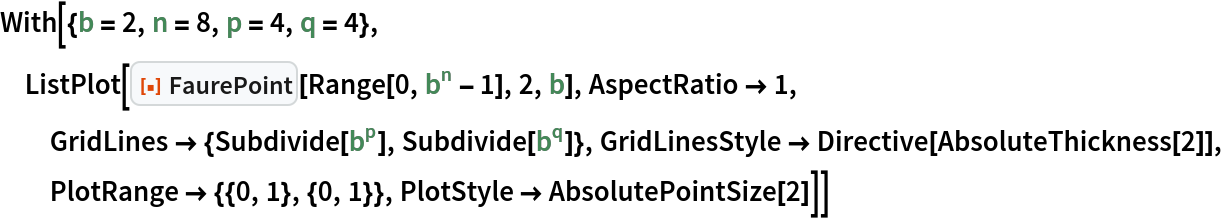Out=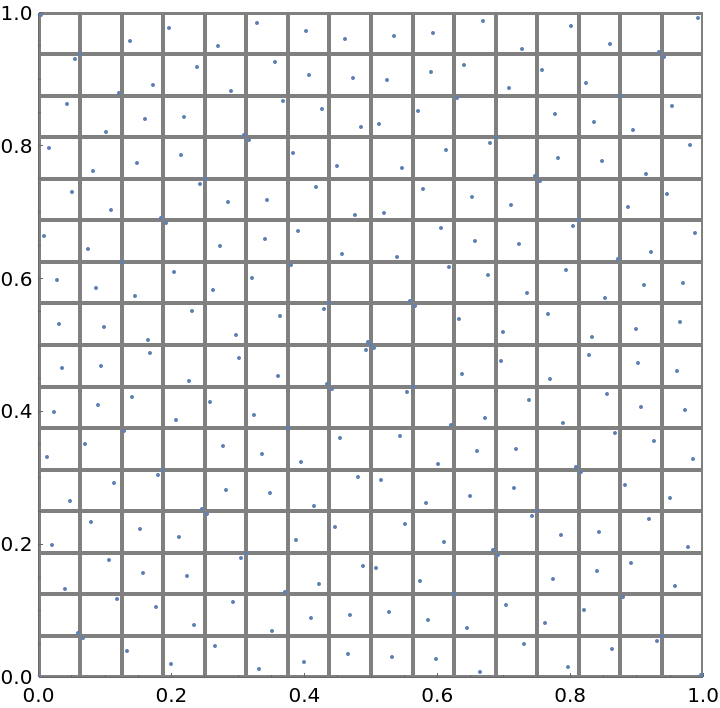## Version History

• 1.0.0 – 01 June 2021